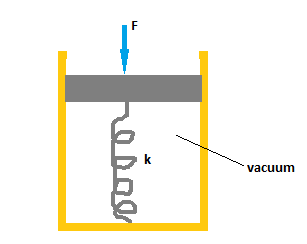# The spring of the pressure gauge shown in the figure below has a force constant of 1000 N/m, and...

## Question:

The spring of the pressure gauge shown in the figure below has a force constant of 1000 N/m, and the piston has a diameter of 1.00 cm. As the gauge is lowered into water in a lake, what change in depth causes the piston to move in by 0.440 cm?## Force on a Spring:

The force on a spring follows Hooke's law. This means that the force is proportional to the displacement by which the spring is contracted or stretched by a constant known as the spring constant. This constant describes the stiffness of a spring.

Become a Study.com member to unlock this answer! Create your account

The force needed to move the piston at that distance is given as:

{eq}F = kx \\ F = (1000)(4.4\times10^{-3}) \\ F = 4.4 \ N {/eq}

The pressure...Hooke's Law & the Spring Constant: Definition & Equation

from

Chapter 4 / Lesson 19
201K

After watching this video, you will be able to explain what Hooke's Law is and use the equation for Hooke's Law to solve problems. A short quiz will follow.